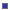# WENO SchemelEssentially non-oscillatory (ENO) and Weighted ENO (WENO) are finite difference or finite volume schemes. The first ENO scheme is constructed by Harten et. al. in 1987.  The first WENO scheme is constructed in 1994 by Liu,Osher and Chan  for a third order finite volume version. In 1996, third and fifth order finite difference WENO schemes in multi space dimensions are constructed by Jiang and Shu, with a general framework for the design of smoothness indicators and nonlinear weights. A key idea in WENO schemes is a linear combination of lower order fluxes or reconstruction to obtain a higher order approximation.  Both ENO and WENO schemes use the idea of adaptive stencils to automatically achieve high order accuracy and non-oscillatory property near discontinuities.  or the system case, WENO schemes based on local characteristic decompositions and  flux splitting to avoid spurious oscillatory. Why is the  WENO scheme  Important?  ENO and WENO schemes are designed for problems with piecewise smooth solutions containing discontinuities. The key idea lies at the approximation level, where a nonlinear adaptive procedure is used to automatically choose the locally smoothest stencil, hence avoiding crossing discontinuities in the interpolation procedure as much as possible. ENO and WENO schemes have been quite successful in applications, especially for problems containing both shocks and complicated smooth solution structures, such as compressible turbulence simulations and aeroacoustics. ˇˇWENO scheme for convection-dominated problemsHamilton-Jacobi equationsWENO scheme in other applicationImages of Compressible fluid flowImages of Hamilton-Jacobi equationsImages of other application

People